# Integration by parts

## Summary and examples

Finding a primitive function is almost impossible. In general, finding the primitive is mostly very difficult or even impossible.
In the topic Integration of standard functions we looked at the following procedure: first look in the table of standard functions. If this fails, try to adjust the integrand and check whether the table can now be used. If this is not the case we try substitution as a possible method.
However, there are more options and one is integration by parts. This method is related to the product rule in differentiation.

Suppose we want to differentiate the product of two functions. We apply the product rule:We can write this expression as: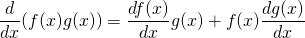When we multiply the left- and right-hand side with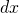(mathematically not completely correct), we get:or: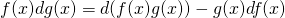We integrate left- and right-hand side: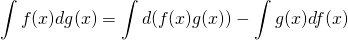and get the following expression: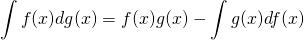To apply integration by parts it is necessary to recognize the relation above. That is certainly difficult, but with some practice, not really a problem.

##### Example 1

We begin with the following integral: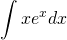We do not recognize the rule above, but we can do something about it.

We can write the derivative of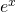as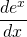which is equal to:After cross-multiplication we get: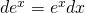Now we can write the integral as: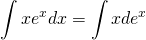According to the rule of integration by parts we can write this as (if we recognize the following relations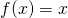and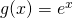):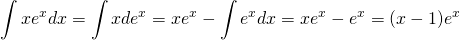Verify the result.

##### Example 2

The following integral can be solved applying the rule of integration by parts: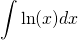At first sight it seems that we do not have a product of two functions, but we can write: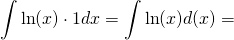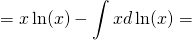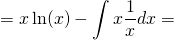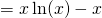Again we can verify the result by differentiation.

0